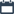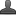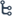## Saturday, October 17, 2020

•October 17, 2020
••, ,
•No comments

## Introduction to Real numbers (10th class )Chapter-1,Notes,Definition,concept,important points

Hi friends and my dear students! In this post, I have covered Andhra Pradesh class -10 Introduction to Real numbers (chapter-1) important points After Reading Mathematics Real numbers (10th class )  Notes With important points, please do share it with your friends. You can Learn Maths for All Classes here.Key concepts:

*The collection of counting numbers are called natural numbers. Set of natural numbers are denoted by 'N'

N={1,2,3,..}

* The collection of natural numbers includes zero are called whole numbers. Set of whole numbers is denoted by W'

W=(0} U { 1,2,3,.. }

= {0,1,2,3,. }

* The collection of whole numbers includes negative numbers are called integers.

Set of integers are denoted by Z' or I

Z={...3,-2,-1,} U {0,1,2,3,.... }

= {.. 3,-2,-1,0,1,2,3,... }

Note: 1) The number O' is neither positive nor negative

2) There is no integer between any two consecutive integers.

Lemma: Lema is a proven statement used for proving another statement Dividend = Divisor x quotient + remainder Euclid's division lemma: for a pair of given positive integers 'a' and b' there exist unique pair of integers q' and 'r' such that a=b q+ r where o ≤ r<b.

Eg: Given positive integers 17 and 6

Let a=17 and b=6

17 = 6x2+5 where 0≤5< 6

Steps to obtain HCF  by  Euclid's division lemma.

Step 1: Consider two positive integers 'a' and b' such that a>b

Step 2: by Euclid's lemma. To find whole numbers 'q' and 'r' such that a=b q+ r

Step 3: If 'r'=0 then b' is the HCF of a and b Step 4 If r + 0 then consider the division of b with the remainder r, continue this process till the remainder becomes zero

Eg:- Find HCF of 972 and 21 let a=972, b=21

by using Euclid's division lemma,

972=21 x 46+6

Here remainder is 6

By Euclid's division lemma

21= 6x3+3

Here remainder is 3.

By Euclid's division lemma,

6 =3x2+0

Now remainder is 'O' .

HCF of 972 and 21 is 3

Note: Euclid's division lemma is stated for only positive integers but it can be extended for all integers except 'O'

Prime number: A natural number N>1have exactly two distinct factors (1 and itself) are called prime numbers.

Eg: 2,3,5,7,11,13,..

Composite number: Natural number N(>1) have more than two factors are called composite numbers

Eg:- 4,6,8,9,10,....

Note: 1) There are infinite primes and composite numbers

2) 1 is neither prime nor composite

3) 2' is even prime

Co-prime: Two numbers are said to be co-primes it their HCF in 1

Ex: (2,3), (4,5)....

Factor: If a number a divides another number b exactly then 'a' is a factor of b'

Eg:- 2 divides 6 exactly so 2 is a factor of 6

Also Check

## Trigonometry Do This & Try this solution

SSC(10th class) Trigonometry Exercise - 11.1 Solutions

FUNDAMENTAL THEOREM OF ARITHMETIC: Every composite number can be expressed as a product of primes and this factorisation is unique.

Eg:- 24= 2 x 2 x 2 x 3.

To obtain LCM and HCF of given number by prime factorization method.

Step 1: Let us consider given numbers be 'a' and b'

Step 2: Express a and b as product of prime powers

Step 3: HCF=product of the smallest power of each common prime factors

LCM=product of the greatest power of each prime factors of the numbers

Rational numbers:

The collection of numbers which are in the form p/q where p and q are integers and q≠ 0 are called rational numbers set of rational numbers is denoted by Q.

Eg:- 3 /2 ,-5 ,1/2

Every rational number can be written in the form of terminating decimal or non-terminating repeating decimals.

Between any two rationals there exist infinite rational numbers

A rational number between any two rational number a and b is a+b/2

Terminating decimals in rational numbers: Let x=p/q be a rational number such that the prime factorisation of is of the form 2n x 5m where n and m are non-negative integers. Then x has decimal expansion which terminates.

Eg:- x=3/20=p/q

Q=20=22 x 5

Which is the  form of   2n x 5m

3/20 is terminating decimals

Non terminating, recuring decimals in rational numbers: Let x=p/q be a rational number, such that the prime factorisation of q is not in the form of 2n x 5m where n and m are non-negative integers. Then x has a decimal expansion, which in non-terminating repeating.

Eg:-1)  x=23 /35=p/q

q= 35 = 5' x7 which is not in the form of 2n x 5m

23/35  is non-terminating repeating decimal

2) x= 1/7 =p/q

Q=7 which not in the form of 2n x 5m

1/7= 0.142857.... is a non-terminating repeating decimal.

Irrational number: Number which cannot be written in the form of  p/q are called irrational numbers. the set of irrational numbers are denoted by Q or S.

Eg: 0.101001000...,1.256789124569....

Every non-perfect square number is an irrational number.

Eg:- V2, 3, V6,.

Note:-1) 7 is an irrational number

2) An irrational number between a and b is√ab

Ex: irrational number between 2 and 3 is 2x3 = 6

Properties:- 1) Sum of a rational number and an irrational number is an irrational number Eg:- 2+3,5+7.

2)Difference of a rational and an irrational number is an irrational number

Eg;4 -V5, 4-V11

3) Product of a non zero rational and an irrational numbers is an irrational number

Eg:- 53,67 ,.. ...

4) Quotient of a non zero rational and  an irrational number is an irrational number

Eg:- 5/3,7/4

5)  the sum of the two irrational numbers need not be irrational.

Eg:- a = 3- 2,b = 3+ V2

Then a+b=3-V2 +3+ V2

= 6 (rational)

6) The product of two irrational numbers need not be irrational,

Eg:- a = 5,b =2V125

Then  a x b= 5 x 2125

= 2x5x 5

= 50 (rational)

Real numbers: The set of rational and irrational numbers together are called real numbers. set of real numbers are denoted by R=QUQ'

Logarithm: Logarithmic form and exponent form are two sides of the same coin. ie) every logarithmic form can be written in the exponent form and vice versa. The theory of logarithm is obtained from the theory of indices.

If a>1. Then ax increases as x increases. and If a<1, then ax decreases as x decreases.

Eg:- 2x increases if x increases

(1/2)x decreases if x decreases.

Hence loga x is an increasing function if a>1 and loga x is a decreasing function if a<1

Logarithms are used for all sorts of

calculations in engineering, science, business and economics.

Defiinition:- If ax = N then x = log when (a # 1) > 0 and N>0 for some a,N  R.

Eg:- log25, log10 100 There are two systems of logarithms.

1) Common logarithms (or) briggs logarithms

2)  2) Natural logarithms (or) Naperian logarithms.

Common Logarithms: Logarithms to the base '10' are called common logarithms.

Eg:- log1050, log10 100

Natural logarithms: Logarithms to the base 'e' are called as natural logarithms. Eg:- logex, loge 5

Here e is an irrational number also called exponential number the value of e=2.718 (Approximately)

Laws of logarithms:

First law: loga xy = loga x+ logay where x,y and 'a' are positive real numbers and a≠1 Proof:- Let logax, = m

and logay,  = n

By definition; x= an..    (1)

y = am.... (2)

By multiplying 1 & 2; xy = an x am

Xy =  am+n

loga xy = m+n

loga xy = loga x+ logay

hence proved

2) loga x/y = loga x-logay

3) loga 1= 0

4) alogam = m

loga xm =mlogax

characteristic and Mantissa: Consider a number N>0. Then let the value of log10N consist of two parts One a integral part, The other a proper fraction. The integral part in called the characternstic and the fractional (or) decimal part is called the mantissa. The mantissa is  always lie between 0 and 1

Eg:- log1015 = 1.176 Here 1 is characteristic 0.176 is mantissa

Note:-1) Characteristic of 'n' digited number is n-1

2) If the characteristic is n then the number of digits is n+1